# Geometry Formulas for Area of the Triangle for CAT Exam

Geometry Formulas for Area of the Triangle for CAT Exam
4.7 (93.33%) 12 votes

There are many formulas to calculate the area of a triangle. Some formulas are very frequently used and some are used specifically for certain types of questions. A student should not only remember those formulas but also know where to apply them.

In this article will discuss all the formulas for finding the area of a triangle. And, as and when required, we will understand the application of that formula with the help of questions which have appeared in CAT and other competitive exams.

The formula for the area of triangles can be categorized as:

• General: These formulas are applicable for all types of triangles.
• Specific:  These formulas are applied only to a specific kind of triangle.

### General Formulas to calculate the area of a triangle

Let us learn all general formulas to find the area of a triangle.1. Area of a triangle when the base of the triangle and the corresponding height is given:

This is the most frequently used formula.

Area of the triangle= $\frac{1}{2} \times base \times height$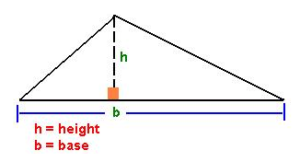Question: Euclid has a triangle in mind, its longest side has length 20 and another of its sides has length 10. Its area is 80. What is the exact length of its third side? [CAT 2001]

1. $\sqrt {260}$
2. $\sqrt {250}$
3. $\sqrt {240}$
4. $\sqrt {270}$

Solution: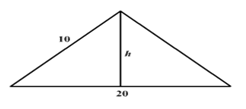Let the perpendicular on the longest side from the other vertices be h.

Therefore, $\frac{1}{2} \times 20 \times h = 80$ i.e., h = 8

The perpendicular has two triangles on its two sides. On its left, there is one with a hypotenuse of 10. If the two sides are 10 and 8, the third one must be 6.

The base of the other triangle is 20 – 6 = 14

The two sides being 8 and 14, the hypotenuse must be $\sqrt{{{14}^{2}}-{{8}^{2}}}=\sqrt{260}$

Hence, the correct answer is option (a)

1. When all the three sides of the triangle are known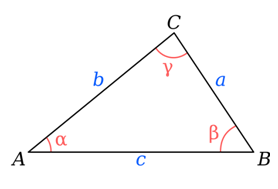Area of the triangle= $\sqrt {(s – a)(s – b)(s – c)}$ , where $s = \frac{{a + b + c}}{2}$ is the semi perimeter of the triangle. This is also known as Heron’s formula.

Note: This formula is very calculation intensive. We deliberately try to avoid using this formula unless we are left with no other options.

1. When a circle is inscribed inside a triangle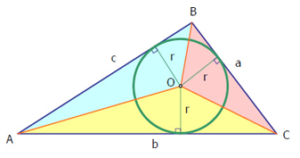This is a very important case for any competitive exam. Many questions have appeared in the exams which can be solved by applying this direct formula. It is also worthwhile to go through the derivation of this formula, as the method of derivation at times is used to solve some of the geometry questions.

Area of the triangle=s×r, where s is the semiperimeter of the triangle and r, is the radius if the inscribed circle.

Question: Find the in-radius of a triangle with side lengths 13, 14, and 15 cms.

Solution:

Semi perimeter (s) = (13+14+15)/2 = 21.

Area of the triangle by using Heron’s formula = $\sqrt {21 \times 8 \times 7 \times 6} = 84$

Also, the area = rxs = 84

Or, rx21=84 hence, in-radius = 4.

Note: The inscribed circle is called in-circle, and its radius is called in-radius.

1. When a triangle is inscribed inside a circle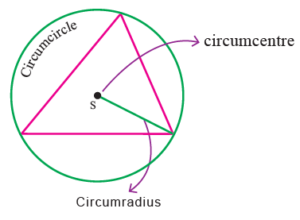Area of the triangle= $\frac{{abc}}{{4R}}$, where R is the radius of the circle

Note: The circle is called circum-circle, and the radius R is called circum-radius

1. When two sides and an including angle is known

Area of the triangle = $\frac{1}{2}ab\sin \theta$ where, a and b are the sides and $\theta$ is the angles between them.

Question: The internal bisector of an angle A in a triangle ABC meets the side BC at point D. AB = 4, AC = 3 and A = 600. Then what is the length of the bisector AD? [CAT 2002]
1. $12\sqrt 3 /7$
2. $12\sqrt {13} /7$
3. $4\sqrt {13} /7$
4. $4\sqrt 3 /7$

Solution: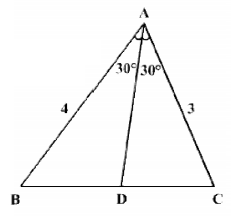In a triangle with sides a and b and the included angle, the area is given by

Area = ab(sinC)/2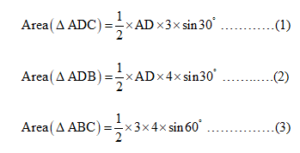(1)+(2)=(3)

i.e., $AD = \left( {\frac{3}{2} + \frac{4}{2}} \right) = 12 \times \frac{{\sqrt 3 }}{2}$

$\Rightarrow AD = \frac{{12\sqrt 3 }}{7}$

Hence, option (a)

There are many other general formulae to calculate the area. However, those formulas require from higher concepts of trigonometry.  We can safely ignore them for CAT exam.

### The specific formula for the area of a triangle

• Area of equilateral triangle = $\frac{{\sqrt 3 }}{2}{a^2}$ , where ‘a’ is the side of the equilateral triangle.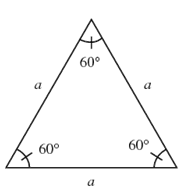• Area of isosceles triangle = $\frac{1}{2} \times a \times \sqrt {{b^2} – \frac{{{a^2}}}{4}}$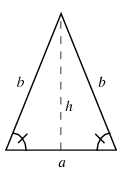Where b is the measure of the equal sides of an isosceles triangle and a is the base of the isosceles triangle.